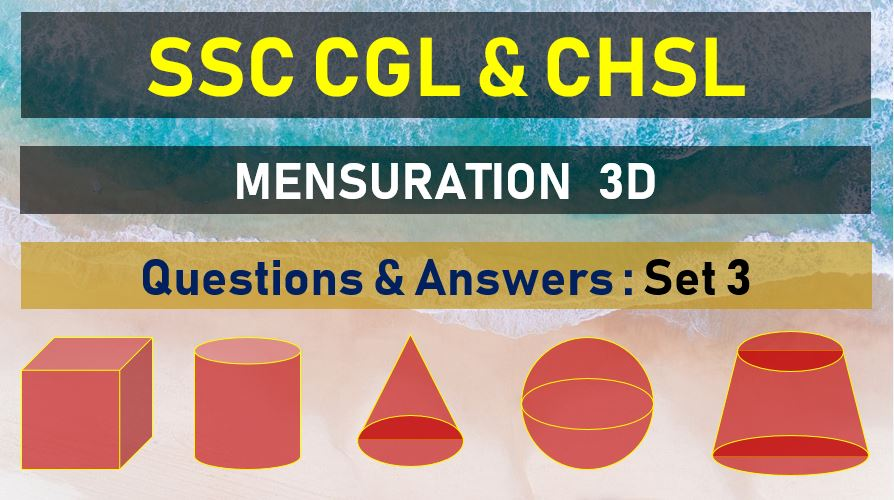Tuesday, June 22, 2021
Home > mensuration > SSC CGL Mensuration 3D Questions Answers Set 3

# SSC CGL Mensuration 3D Questions Answers Set 3SSC Mensurations 3D
Surface Area & Volume Questions Sets
Set 1Set 2Set 3Set 4Set 5
Set 6Set 7Set 8Set 9Set 10

SSC CGL Mensuration 3D Questions Answers Set 3: Ques No 1

A corn cob, shaped somewhat like a cone, has the radius of its broadcast end as 2.1 cm and length (height) as 20 cm. If each 1 cm2 of the surface of the cob carries an average of four grants, find how many grains (approximately) you would find on the entire cob.

Options:

A. 511

B. 531

C. 551

D. 571

Answer: B

Solution:

SSC CGL Mensuration 3D Questions Answers Set 3: Ques No 2

The radius of the base of a conical tent is 7 m. The tent is 24 m high. Find the cost of the canvas required to make the tent, if one square meter of canvas costs Rs. 180.

Options:

A. Rs. 99000

B. Rs. 98000

C. Rs. 95000

D. Rs. 97000

Answer: A

Solution:

SSC CGL Mensuration 3D Questions Answers Set 3: Ques No 3

Base of a right prism is a rectangle, the ratio of whose length and breadth is 3:2 if the height of the prism is 12 cm and total surface area is 288 sq. cm, the volume of the prism is :

Options:

A. 288 cm3

B. 290 cm3

C. 286 cm3

D. 291 cm3

Answer: A

Solution:

SSC CGL Mensuration 3D Questions Answers Set 3: Ques No 4

The base of a right prism is a trapezium whose lengths of two parallel sides are 10 cm and 6 cm and distance between them is 8 cm , its volume is

Options:

A. 300 cm3

B. 300.5 cm3

C. 320 cm3

D. 310 cm3

Answer: C

Solution:

SSC CGL Mensuration 3D Questions Answers Set 3: Ques No 5

A right prism has triangular base. If v be the number of vertices, e be the number of faces of the prism. The value of v + e –f/2 is

Options:

A. 2

B. 4

C. 5

D. 10

Answer: C

Solution:

SSC CGL Mensuration 3D Questions Answer Set 3: Ques No 6

Base of a prism of height 10 cm square. Total surface area of the prism is 192 sq. cm . the volume of the prism is

Options:

A. 120 cm3

B. 640 cm3

C. 90 cm3

D. 160 cm3

Answer: D

Solution:

SSC CGL Mensuration 3D Questions Answer Set 3: Ques No 7

A right prism has a triangular base whose sides are 13 cm, 20 cm and 21cm, if the altitude of the prism is 9 cm, then its volume is

Options:

A. 1143 cm3

B. 1314 cm3

C. 1413 cm3

D. 1134 cm3

Answer: D

Solution:

SSC CGL Mensuration 3D Questions Answer Set 3: Ques No 8

Base of a right pyramid is a square of side 10 cm. If the height of the pyramids 12 cm, then its total surface area is

Options:

A. 360 cm2

B. 400 cm2

C. 460 cm2

D. 260 cm2

Answer: A

Solution:

SSC CGL Mensuration 3D Questions Answer Set 3: Ques No 9

The total surface area of a regular triangular pyramid with each edges of length 1 cm is?

Options:

A. 4/2(√2) cm2

B. √3 cm2

C. 4 cm2

D. 4√3 cm2

Answer: B

Solution:

SSC CGL Mensuration 3D Questions Answer Set 3: Ques No 10

If the base of right prism remains same and the lateral edges are halved then it s volume will be reduced by

Options:

A. 33.33%

B. 50%

C. 25%

D. 66%

Answer: B

Solution:

SSC Mensurations 3D
Surface Area & Volume Questions Sets
Set 1Set 2Set 3Set 4Set 5
Set 6Set 7Set 8Set 9Set 10
error: Content is protected !!